Next: FT OF RANDOM NUMBERS Up: My rise-time proof of Previous: My rise-time proof of

### Proof by way of the dual problem

Although the Z-transform method is a great aid in studying cases where divergence (as 1/t) plays a role, it has the disadvantage that it destroys the formal interchangeability between the time domain and the frequency domain. To take advantage of the analytic simplicity of the Z-transform, we consider instead the dual to the rise-time problem. Instead of a signal whose square vanishes at negative time, we have a spectrum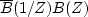that vanishes at negative frequencies. We measure how fast this spectrum can rise after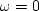.We will find this time interval to be related to the time durationof the complex-valued signal bt. More precisely, we now define the lowest significant frequency component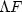in the spectrum, analogously to (3), as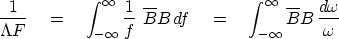(4)
where we have assumed the spectrum is normalized, i.e., the zero lag of the auto-correlation of bt is unity. Now recall the bilinear transform, equation (), which representsas the coefficients of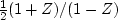, namely,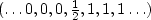.

The pole right on the unit circle at Z = 1 causes some nonuniqueness. Becauseis an imaginary, odd, frequency function, we will want an odd expression (such as on page) to insert into (4):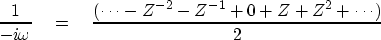(5)
Using limits on the integrals for time-sampled functions and inserting (5) into (4) gives(6)
Let st be the autocorrelation of bt. Since any integral around the unit circle of a Z-transform polynomial selects the coefficient of Z0 of its integrand, we have(7) (8)
The height of the autocorrelation has been normalized to s0=1. The sum in (8) is an integral representing area under the |st| function. So the area is a measure of the autocorrelation width.Thus,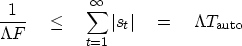(9)

Finally, we must relate the duration of a signalto the duration of its autocorrelation.Generally speaking, it is easy to find a long signal that has short autocorrelation. Just take an arbitrary short signal and convolve it using a lengthy all-pass filter. Conversely, we cannot get a long autocorrelation function from a short signal. A good example is the autocorrelation of a rectangle function, which is a triangle. The triangle appears to be twice as long, but considering that the triangle tapers down, it is reasonable to assert that the's are the same. Thus, we conclude that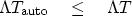(10)
Inserting this inequality into (9), we have the uncertainty relation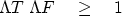(11)

Looking back over the proof, I feel that the basic time-bandwidth idea is in the equality (7). I regret that the verbalization of this idea, boxed following, is not especially enlightening. The inequality arises from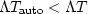,which is a simple idea.

 The inverse moment of the normalized spectrum of an analytic signal equals the imaginary part of the mean of its autocorrelation.

## EXERCISES:

1. Consider B(Z) = [1 - (Z/Z0)n]/(1 -Z/Z0) as Z0 goes to the unit circle. Sketch the signal and its squared amplitude. Sketch the frequency function and its squared amplitude. Chooseand.
2. A time series made up of two frequencies can be written as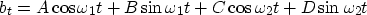Given,, b0, b1, b2, and b3, show how to calculate the amplitude and phase angles of the two sinusoidal components.Next: FT OF RANDOM NUMBERS Up: My rise-time proof of Previous: My rise-time proof of
Stanford Exploration Project
10/21/1998